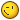# P2 gravity Center ?

## Recommended Posts

Hi !!

I'm on a space game using P2, and i'm trying to create a center of gravity for the planets, to be more explicit, i want all the planets attract the spaceship bu the planet center...

If you don't understand or if i did too many error with my worlds, i could try to re explain better ^^!

##### Share on other sites

before i start fantasizing about possible solutions for your problem (because dot gravity is nothing p2 can do atm as far as i know) i need to ask more questions.

will there be more than one planet attracting the spaceship at a time?

should gravity lose it's effect with more distance to the planet?

how many objects are usually affected by the planets gravity? only the spaceship?

##### Share on other sites

before i start fantasizing about possible solutions for your problem (because dot gravity is nothing p2 can do atm as far as i know) i need to ask more questions.

will there be more than one planet attracting the spaceship at a time?

should gravity lose it's effect with more distance to the planet?

how many objects are usually affected by the planets gravity? only the spaceship?

Hi !

For now, i did that :

`game.physics.p2.gravity.y = planete.body.y - fusee.body.y;game.physics.p2.gravity.x = planete.body.x - fusee.body.x;`

But it's bugging a little and i'm looking for a better solution !

1- No

2- Yes Sure !

3- For now, only the spaceship yes##### Share on other sites

it's space.. so you could stop using gravity and instead use forces....

you could use this function (give it your spaceship and the planet) to get the distance between 2 objects and then calculate the speed based on the returned distance

`function distanceBetween(spriteA,spriteB){    var dx = spriteA.body.x - spriteB.body.x;  //distance ship X to planet X    var dy = spriteA.body.y - spriteB.body.y;  //distance ship Y to planet Y    var dist = Math.sqrt(dx*dx + dy*dy);     //pythagoras ^^  (get the distance to each other)    return dist;}`

define speed (force)

`if (distanceBetween(ship,planet) < 300) { speed = 30;}else if (distanceBetween(ship,planet) < 600) { speed = 20;}else if (distanceBetween(ship,planet) < 900) { speed = 10;}`

and then call this function to create a force  into the direction of the planet

`function accelerateToObject(obj1, obj2, speed) {    if (typeof speed === 'undefined') { speed = 20; }    var angle = Math.atan2(obj2.y - obj1.y, obj2.x - obj1.x);    obj1.body.rotation = angle + game.math.degToRad(180);  // correct angle if wanted    obj1.body.force.x = Math.cos(angle) * speed;    // accelerateToObject     obj1.body.force.y = Math.sin(angle) * speed;}`
##### Share on other sites

http://phaser.io/sandbox/edit/awDIvALb

phaser sandbox.. hope it works##### Share on other sites

it's space.. so you could stop using gravity and instead use forces....

you could use this function (give it your spaceship and the planet) to get the distance between 2 objects and then calculate the speed based on the returned distance

`function distanceBetween(spriteA,spriteB){    var dx = spriteA.body.x - spriteB.body.x;  //distance ship X to planet X    var dy = spriteA.body.y - spriteB.body.y;  //distance ship Y to planet Y    var dist = Math.sqrt(dx*dx + dy*dy);     //pythagoras ^^  (get the distance to each other)    return dist;}`

define speed (force)

`if (distanceBetween(ship,planet) < 300) { speed = 30;}else if (distanceBetween(ship,planet) < 600) { speed = 20;}else if (distanceBetween(ship,planet) < 900) { speed = 10;}`

and then call this function to create a force  into the direction of the planet

`function accelerateToObject(obj1, obj2, speed) {    if (typeof speed === 'undefined') { speed = 20; }    var angle = Math.atan2(obj2.y - obj1.y, obj2.x - obj1.x);    obj1.body.rotation = angle + game.math.degToRad(180);  // correct angle if wanted    obj1.body.force.x = Math.cos(angle) * speed;    // accelerateToObject     obj1.body.force.y = Math.sin(angle) * speed;}`

Amazing.. !

My mistakes was to use gravity instead of force ! It's so cool !

Thank you man !!

##### Share on other sites

• 1 year later...

Yes, thank you so much for this very illustrative sample